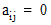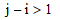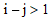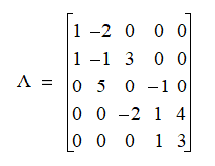# NMath User's Guide

17.6 Tridiagonal Matrices (.NET, C#, CSharp, VB, Visual Basic, F#)

A tridiagonal matrix is a matrix which has all its non-zero entries on the main diagonal, the superdiagonal, and the subdiagonal. That is,wheneveror. For example, this is a 5 x 5 tridiagonal matrix:Tridiagonal matrices often occur in one-dimensional problems and at an intermediate stage in the process of finding eigenvalues.

NMath provides tridiagonal matrix classes for four datatypes: single- and double-precision floating point numbers, and single- and double-precision complex numbers. The classnames are , , , and .

For efficiency, zero elements outside the main diagonal, superdiagonal, and subdiagonal are not stored. A tridiagonal matrix is a special case of a banded matrix where the upper and lower bandwidths are one, and the storage scheme is the same as for a banded matrix (Section 17.5).

Top

Top